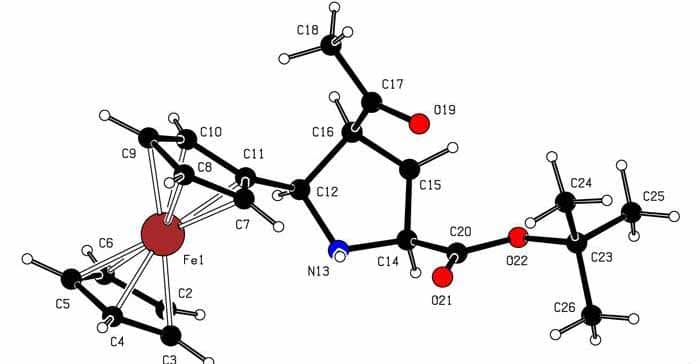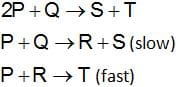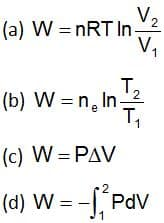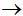Engineering Entrance Sample Papers

SRMJEEE Chemistry Sample PaperThis sample practice paper consist 35 questions on chemistry as per exam pattern of SRMJEE Entrance Exam.

Ques. Which one of the following pairs of solutions can we expect to be isotonic at the same temperature
(a) 0.1 M urea and 0.1 M NaCl
(b) 0.1 M urea and 0.2 MMgCl2
(c)  0.1 M NaCl and 0.1 M Na2SO4
(d) 0.1 M Ca(NO3)2 and 0.1 M Na2SO4
Ans. (d)

Ques. Aniline reacts with which of these to form Schiff base
(a) Acetic acid
(b) Benzaldehyde
(c) Acetone
(d) NH3
Ans: (b)

Ques. Which of the following contains a coordinate covalent bond
(a) N2H5+
(b) BaCl2
(c) HCl
(d) H2O
Ans. (a)

Ques. The unit cell of a  lattice
(a) Is body centred cube
(b) Has 3Na+ ions
(c) Has 4NaCl units
(d) Is electrically charged
Ans. (c)

Ques. What is kinetic energy of 1g of O2 at 47oC
(a) 1.24 x 102 J
(b) 2.24 x 102 J
(c) 1.24 x 103 J
(d) 3.24 x 102 J
Ans. (a)

Ques. The mechanism for the reaction is given belowThe rate law expression for the reaction is
(a) r = k [P]2[Q]
(b) r = k[P][Q]
(c) r = k[A][R]
(d) r = k[P]2
Ans. (b)

Ques. The volume of 1 g each of methane (CH4), ethane (C2H6), propane (C3H8) and butane (C4H10) was measured at  350  K  and  1 atm. What is  the  volume  of butane
(a) 495 cm3
(b) 600 cm3
(c) 900 cm3
(d) 1700 cm3
Ans. (a)

Ques. For a sparingly soluble salt ApBq, the relationship of its solubility product (LS) with its solubility (S) is
(a) Ls = Sp + q . pp . qq
(b) Ls = Sp + q . pq . qp
(c) Ls = Spq . pp . qq
(d) Ls = Spq . (p.q)p+q
Ans. (a)

Ques. The ONO angle is maximum in
(a) NO3
(b) NO2
(c) NO2
(d) NO2+
Ans. (d)

Ques. When sulphur is boiled with Na2SO3 solution, the compound formed is
(a) Sodium sulphide
(b) Sodium sulphate
(c) Sodium persulphate
(d) Sodium thiosulphate
Ans. (d)

Ques. Ammonia is a Lewis base. It forms complexes with cations. Which one of the following cations does not form complex with ammonia
(a) Ag+
(b) Cu++
(c) Cd++
(d) Pb++
Ans. (d)

Ques. Which expression is correct for the work done in adiabatic reversible expansion of an ideal gasAns. (b)

Ques. An organic compound contains 49.3% carbon 6.84% hydrogen and its vapour density is 73. Molecular formula of the compound is
(a) C3H5O2
(b) C6H10O4
(c) C3H10O2
(d) C4H10O2
Ans. (b)

Ques. In Wurtz reaction, the reagent used is
(a) Na
(b) Na / liquid NH3
(c) Na / dry ether
(d) Na / dry alcohol
Ans. (c)

Ques. Which of the following is not correct for N2O
(a) It is called laughing gas
(b) It is nitrous oxide
(c) It is not a linear molecule
(d) It is least reactive in all oxides of nitrogen
Ans. (c)

Ques. Replacement of Cl of chlorobenzene to give phenol requires drastic conditions but chlorine of 2, 4-dinitrochlorobenzene is readily replaced because
(a)NO2 make ring electron rich at ortho and para
(b) NO2 withdraws efrom meta position
(c) denotes e at meta position
(d) NO2 withdraws efrom ortho/para positions
Ans. (d)

Ques. Which of these do not undergo Wurtz reaction
(a) C2H5F
(b) C2H5Br
(c) C2H5Cl
(d) C2H5I
Ans. (a)

Ques. Which of the following cannot be reduced by H2O2
(a) Ag2O
(b) Fe3+
(c) Acidified KMnO4
(d) Acidified K2Cr2O7
Ans. (b)

Ques. The decay constant of a radioactive element is 3 x 10–6 min–1.  Its half-life is
(a) 2.31 x 105 min
(b) 2.31 x 106 min
(c) 2.31 x 10–6 min
(d) 2.31 x 10–7 min
Ans. (a)

Ques. A white substance having alkaline nature in solution is
(a) NaNO3
(b) NH4Cl
(c) Na2CO3
(d) Fe2O3
Ans. (c)

Ques. The vapour density of completely dissociated NH4Cl would be
(a) Slight less than half that of NH4Cl
(b) Half  that of NH4Cl
(c) Double that of NH4Cl
(d) Determined by the amount of solid NH4Cl  in the experiment
Ans. (b)

Ques. Which one of the following pairs of solutions can we expect to be isotonic at the same temperature
(a) 0.1 M urea and 0.1 M NaCl
(b) 0.1 M  urea and 0.2 M MgCl2
(c) 0.1 M NaCl and 0.1 M Na2SO4
(d) 0.1 M Ca (NO3)2 and 0.1 M Na2SO4
Ans. (d)

Ques: Molecular formula of chloropicrin is
(a) CHCl3NO2
(b) CCl3NO3
(c) CCl2NO2
(d) CCl3NO2
Ans: (d)

Ques. If the Kb value in the hydrolysis reaction B+ + H2O ⇄ BOH + H+ is 1.0 x 10–6, then the hydrolysis constant of the salt would be
(a) 1.0 x 10–6
(b) 1.0 x 10–7
(c) 1.0 x 10–8
(d) 1.0 x 10–9
Ans. (c)

Ques. 10 dm3 of N2 gas and 10 dm3 of gas X at the same temperature contain the same number of molecules. The gas X is
(a) CO
(b) CO2
(c) H2
(d) NO
Ans. (a)

Ques. The electrons identified by quantum numbers n and l (i)  (ii)  (iii)  (iv)  can be placed in order of increasing energy from the lowest to highest, as
(a) (iv) < (ii) < (iii) <(i)
(b) (ii) < (iv) < (i) < (iii)
(c) (i) < (iii) < (ii) < (iv)
(d) (iii) < (i) < (iv) < (ii)
Ans. (a)

Ques. The root mean square speeds at STP for the gases H2, N2, O2 and HBr are in the order
(a) H2 < N2 < O2 < HBr
(b) HBr < O2 < N2 < H2
(c) H2 <N2 = O2 < HBr
(d) HBr < O2 < H2 < N2
Ans. (b)

Ques. Arrange NH4+, H­2O, H3O+, HF and OHin increasing order of acidic nature
(a) H3O+ < NH4+ < HF < OH < H2O
(b) NH4+ < HF < H3O+ < H2O < OH
(c) OH < H2O < NH4+ < HF < H3O+
(d) H3O+ > HF > H2O > NH4+ > OH
Ans. (c)

Ques. The pH of 0.1 M solution of the following salts increases in the order
(a) NaCl < NH4Cl < NaCN < HCl
(b) HCl < NH4Cl < NaCl < NaCN
(c) NaCN < NH4Cl < NaCl < HCl
(d) HCl < NaCl < NaCN < NH4Cl
Ans. (d)

Ques. In the Fischer-Tropsch synthesis of petrol….. and ….. are used as the raw materials
(a) H2 ; CO
(b) CH4 ; H2
(c) CH4 ; CH3OH
(d) CH3OH ; CO
Ans. (a)

Ques. Benzene is obtained by
(a) Substitution of three acetylene molecules
(c) Polymerisation of three C2H2 molecules
(d) Condensation of three C2H2 molecules
Ans. (c)

Ques. 0.0833 ml of carbohydrate of empirical formula  contain  of hydrogen. The molecular formula of the carbohydrate is
(a) C5H10O5
(b) C3H4O3
(c) C12H22O11
(d) C6H12O6
Ans. (d)

Ques. When zinc is added to CuSO4 copper gets ppt. due to
(a) Reduction of copper ions
(b) Oxidation of copper ions
(c) Hydrolysis of copper sulphate
(d) Complex formation
Ans. (a)

Ques. PbO2 is
(a) Basic
(b) Acidic
(c) Neutral
(d) Amphoteric
Ans. (d)

Ques. A catalyst is a substance which
(a) Increases the equilibrium concentration of the product
(b) Changes the equilibrium constant of the reaction
(c) Shortens the time to reach equilibrium
(d) Supplies energy to the reaction
Ans. (c)

Ques. For reaction 2A + Bproducts, the active mass of B is kept constant and that of A is doubled. The rate of reaction will then
(a) Increase 2 times
(b) Increase 4 times
(c) Decrease 2 times
(d) Decrease 4 times
Ans. (b)